#### You may also like### I'm Eight

Find a great variety of ways of asking questions which make 8.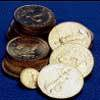### Five Coins

Ben has five coins in his pocket. How much money might he have?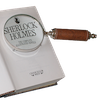### Number Detective

Follow the clues to find the mystery number.

# Which Symbol?

## Which Symbol?

Put in the missing symbols to make these number sentences correct.

Use $+ , -, \times , \div$ and $=$ .

For example: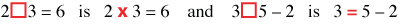Try these: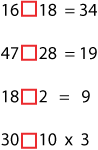All these number sentences below, except two of them, have two solutions.
Can you find the symbols to use?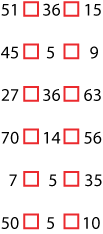Which two number sentences have only one answer?
Can you see why this is so?

### Why do this problem?

This problem is designed to help young learners to use the symbols plus, minus, multiplied by, divided by and equals to, meaningfully, in number statements. Children frequently meet boxes or similar devices to represent numbers but seldom the actual operational symbols. This problem also helps learners understand inverse operations.

### Possible approach

You could start with an example like the ones given at the beginning of the problem.

After this the children could work in pairs on the examples either from the screen or this printed sheet so that they are able to talk through their ideas with a partner.

At the end of the session the group could gather together again and put up their ideas on the board. You should also discuss why four of the double number sentences have two answers and two only have one answer. Can they see why this is so?

This problem could also be used as a people maths activity during an assembly with children standing in line holding cards, thus forming a human equation. The audience can tell the 'symbols' where to stand.

### Key questions

What does this symbol mean?
Which symbol tells you to take away?
What do you have to do to "undo" an addition? What about a subtraction?
What do you have to do to "undo" a multiplication? What about a division?

### Possible extension

Learners could make some more number statements which can be done in more than one way. Can they make one that can be done three or even four ways?

### Possible support

Suggest using counters with the five symbols on them which can be moved around on this sheet.